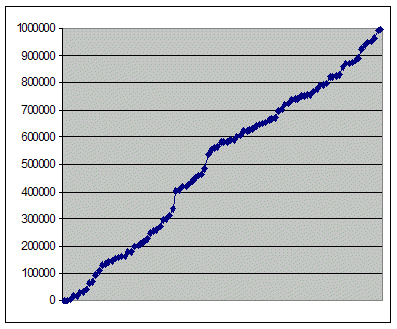# Select 100 random values from a stream / linked list

Here’s an interesting puzzle / interview question that I heard recently.

Problem

You have a either a linked list or a stream containing int values. You don’t know how large the stream / linked list except that it contains 100 or more values. You need to write a method that selects 100 random values from the stream. You have to write the method so that there isn’t a bias towards values at the start, middle or end of the stream / list. In other words the chances of picking a value from anywhere in the stream / list should be roughly the same.

There are some restrictions.
You can’t cache the whole stream (if it is a stream).
Or if it is a linked list – you are only allowed a single pass of the list. You can’t iterate through it once to determine its length.

Solution

The solution is:

1. Select the first 100 values and store them in an array.
2. For each value after the first 100 we select them according to a decreasing probability. If we select a value then we replace a randomly selected value from our existing list.

So values 1-100 have a probability of 1 of being initially selected.
Value 101 has a 100/101 chance of being selected.
Value 102 has a 100/102 chance of being selected.
Value 1050 has a 100/1050 chance… etc.

100 is at the top of the division as this is our value for x – the number of values we are selecting.

You can see that value 101 has a very high chance of being selected. 100/101 in fact. As we are replacing one of our existing values, each of the existing values has a 100/101 chance of remaining after the replacement.

Using this simple logic we can get a random sample of the values even though we don’t know the length of the stream / list.

Code

Here is the C++ code showing how to do this. Also included is a simple test which sends the values 1-1 million to the function. 100 are randomly selected.

After the code is a graph which shows the distribution of the selected values. You can see that the distribution is fairly even showing that the code works. For random number selection code such as this, the easiest way to verify that the code works is to manually view the results in a graph and see if the output looks sensible.

```const int size = 100;
int selected[size];

int count = 0;
void SelectRandomNumbers(int nextNum)
{
if (count < size)
{
selected[count++] = nextNum;
return;
}
double probability = (double)size / (double)(count+1);
double select = (double)rand() / RAND_MAX;
if (select < probability)
{
int randomIndex = RandomNumber(0, size);
selected[randomIndex] = nextNum;
}
++count;
}

// min is inclusive
// max is non-inclusive
int RandomNumber(int min, int max)
{
return (int)((double)rand()	/
(RAND_MAX + 1) * (max - min) + min);
}

///////////////

const int iterations = 1000000;

void RandomSelectionTest()
{
srand((unsigned)time(0));

for (int i=0; i<iterations; ++i)
{
SelectRandomNumbers(i);
}

cout << size <<	" randomly selected ints:" << endl;
PrintIntArray(selected, size);
}

void PrintIntArray(int thearray[], int size)
{
for (int i=0; i<size; ++i)
{
cout << thearray[i] << endl;
}
}
```

Graph

Here are the results. 1 million numbers were passed in. 100 were selected. You can see that the distribution is fairly even which means that the code is doing the correct thing.